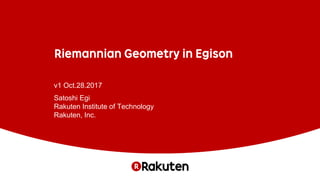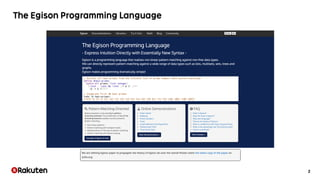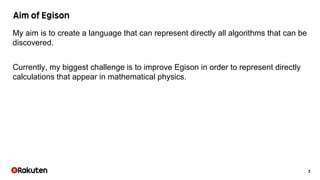Successfully reported this slideshow.

# Riemannian Geometry in Egison×

# Riemannian Geometry in Egison

Most of the technical notations in mathematics and physics are not supported in programming languages.Therefore, we need to translate mathematical expressions to represent them in programming languages.As the result, programs are more complicated than mathematical expressions.This is a big obstacle to cope with problems in mathematics and physics using computers.Egison is a programming language that has been developed to solve this problem.Recently, as part of the solution to this problem, I developed a method for importing tensor index notation into programming with a simple extension of languages.In this lecture, I will show a hint of what kind of ideas are necessary to import mathematical notation into programming by showing this example.

Most of the technical notations in mathematics and physics are not supported in programming languages.Therefore, we need to translate mathematical expressions to represent them in programming languages.As the result, programs are more complicated than mathematical expressions.This is a big obstacle to cope with problems in mathematics and physics using computers.Egison is a programming language that has been developed to solve this problem.Recently, as part of the solution to this problem, I developed a method for importing tensor index notation into programming with a simple extension of languages.In this lecture, I will show a hint of what kind of ideas are necessary to import mathematical notation into programming by showing this example.

### Riemannian Geometry in Egison

1. 1. v1 Oct.28.2017 Satoshi Egi Rakuten Institute of Technology Rakuten, Inc.
2. 2. 2
3. 3. 3 My aim is to create a language that can represent directly all algorithms that can be discovered. Currently, my biggest challenge is to improve Egison in order to represent directly calculations that appear in mathematical physics.
4. 4. 4 Pattern-matching against the wider range of data types. Customizable symbolic computation using Egison pattern-matching. Tensor index notation in programming.
5. 5. 5 https://www.egison.org/math
6. 6. 6 https://www.egison.org/math
7. 7. 7 The theory for investigating a curved space. It is easy for us in the 3 dimensional space to recognize a 2 dimensional curved surface is curved. How about our world? Is it curved for who can recognize the higher dimensional space? https://commons.wikimedia.org/wiki/File:Trian gles_(spherical_geometry).jpg
8. 8. 8 The theory explains the gravity as the curve of 4 dimensional time-space. https://commons.wikimedia.org/wiki/File:Spacetime_lattice_analogy.svg
9. 9. 9 In our recognition, the space-time is flat.
10. 10. 10 But, according to the theory, the space-time is curved.
11. 11. 11 And, things move straight in this space-time.
12. 12. 12 In our recognition, it looks like things are falling.
13. 13. 13 Formulas in Riemannian geometry are represented with partial derivative operator and tensor index notation. We can represent both of them concisely in Egison!
14. 14. 14 Egison program that represents the above formula Formula of Riemann curvature tensor ~: superscript _: subscript
15. 15. 15 Pattern-matching against the wider range of data types. Customizable symbolic computation using Egison pattern-matching. Tensor index notation in programming.
16. 16. 16 Pattern-matching against the wider range of data types. Customizable symbolic computation using Egison pattern-matching. Tensor index notation in programming.
17. 17. 17 Pattern Body Matcher Target
18. 18. 18 are patterns.
19. 19. 19 Pattern-matching against the wider range of data types. Customizable symbolic computation using Egison pattern-matching. Tensor index notation in programming.
20. 20. 20
21. 21. 21 We can apply Egison pattern-matching against math expressions. Math expressions are a multiset of terms. Terms are a multiset of factors. Therefore, Egison pattern-matching is very useful to handle them.
22. 22. 22 Pattern-matching against the wider range of data types. Customizable symbolic computation using Egison pattern-matching. Tensor index notation in programming.
23. 23. 23 Tensor index notation is a popular notation in the field of mathematics and physics.
24. 24. 24 We can control the way for multiplying vectors by indices.
25. 25. 25 We can use tensor index notation to multiply any order of tensors. The tensor index notation is necessary to represent the multiplication of order tensors higher than matrices.
26. 26. 26 In Egison method, we can apply directly both “∂/∂” and “.” functions to tensors. Egison program that represents the above formula Formula of Riemann curvature tensor ~: superscript _: subscript
27. 27. 27 Wolfram program that represents the above formula Egison program that represents the above formula Formula of Riemann curvature tensor
28. 28. 28https://arxiv.org/abs/1702.06343
29. 29. 29
30. 30. 30 https://commons.wikimedia.org/wiki/File:T riangles_(spherical_geometry).jpg
31. 31. 31
32. 32. 32
33. 33. 33
34. 34. 34
35. 35. 35
36. 36. 36
37. 37. 37 https://commons.wikimedia.org/wiki/File:Spacetime_lattice_analogy.svg
38. 38. 38
39. 39. 39 Currently, I am working to represent differential forms, exterior derivative, and Hodge operator directly in Egison. If we realize that, there are the wide range of application, e.g. mathematics, physics, and computer simulation.